# Arecchi¶

## Neurons¶

{Arecchi, 2007a}

Since the axons have lengths between some micrometers in the brain cortex and one meter in the spinal chord, only the electrical propagation assures transmission times of a fraction of a second. The alternative would be a transport by flow as with hormones in the blood, or by molecular diffusion between two cell membranes. The former may require tens or hundreds of seconds; the latter is convenient only for very small separations d, since the diffusion time T scales as $$T = \frac{d^2}{D}$$, where the diffusion constant D for bio-molecules in water is around $$10^{-6} cm^2/sec$$. Thus for d = 1mm, T would be 104 sec (about 3 hours) against an electric transport time d/v = 1ms.

## The homoclinic neuron¶

{Arecchi et al., 1986}

{Allaria et al., 2001}

A dynamical model, which will be presented elsewhere, reproduces with quantitative accuracy all the relevant features above reported and provides a sound basis for a heuristic interpretation of these phenomena as well as a motivation for their widespread occurrence.

{Arecchi et al., 2002}, {Leyva et al., 2003} have 6-equation system; cf. Arecchi04 Fig. 3 which plots x1 X x6

{Arecchi, 2004}

{Arecchi, 2007a}

The most plausible mechanism for it is the chaos due to a saddle focus S bifurcation (Shilnikov chaos) (Shilnikov). Let us see how it occurs (Fig.5).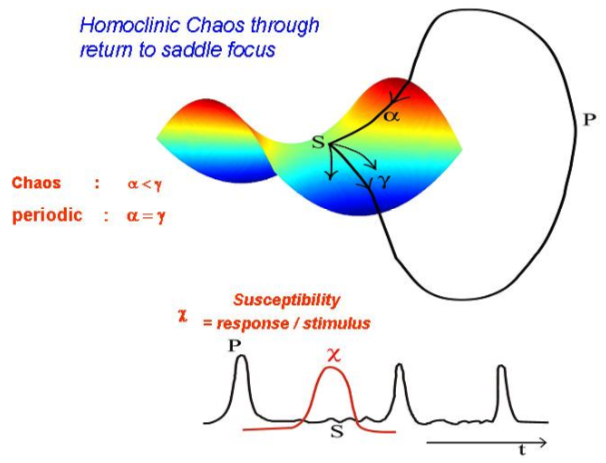Fig. 106 The phase space trajectory escapes from S through the unstable manifold and returns to S through the stable one.¶ Homoclinic chaos through a saddle focus bifurcation {Arecchi, 2007a, Fig. 4}

The trajectory in phase space is a closed one (HC = homoclinic chaos) with a return to S through a stable manifold with contraction rate α and escape through the unstable manifold with expansion rate γ. Chaos requires α<γ. Away from S the motion is regular and gives rise to an identical peak P per turn. The inter-peak interval (ISI) is chaotic due to the variable amount of time spent around S. The HC dynamics has been studied in detail for a CO2 laser (Arecchi et al, 1987,1988); for convenient control parameters, neuron models as Hodgkin-Huxley or Hindmarsh-Rose present HC. {Feudel et al., 2000}.

The qualitative dynamics sketched in Fig. 4 shows that HC occurs under very general assumptions; thus we set aside specific physiological mechanisms and model the individual neuron as an HC system. The susceptibility χ (sensitivity to an external perturbation) is high around S and low everywhere else [] (Arecchi 2004); thus the system is very resilient to uniformly distributed noise. The high χ allows a response correlated in time with an external perturbation. This has been proved by synchronization to a periodic applied signal (Allaria et al) or by mutual synchronization of an array of coupled identical HC systems, modeling the neurons an interacting cortical area {Leyva et al., 2003}.

In fig. 5 I plot the space–time locations of spikes (each one represented as a point) in an array of HC systems with nearest neighbor coupling. They represent a plausible model for a set of coupled neurons. From left to right, we have different degrees of coupling. Full synchronization is reached above a critical coupling strength. By synchronization we do not mean isochronism, which would imply horizontal lines in the plot of fig.5. We have to account for lags in the dynamical coupling; furthermore, in real neuron arrays one should also account for delays in the axonal propagation. Thus, synchronism means that the spikes in neighboring sites are separated by much less than the average ISI; otherwise there would be a spike missing (as the “holes” or defects in the patterns at intermediate couplings). Of course, in a long chain the single spike time separation between the first and the last can be much large than the average ISI; yet the system has a coherent pattern without defects.

## Feature binding¶

{Arecchi, 2007a}

Neural integration consists of a correlation between neurons, even far away from each other, when their receptive fields extract different features of the same object. This correlation (feature binding; see: Singer, Gray, Chawla, Duret) is a collective state with neurons having their spikes synchronized.

Psychophysical studies have shown that the analysis of visual scenes occurs in two phases.

1. First, the elementary features of the objects, as color, motion, contour orientation, are locally detected in parallel.

2. Next, these components are connected to provide a coherent object representation (Gestalt).

More precisely, feature binding denotes how coupled neurons combine external signals with internal memories into new coherent patterns of meaning. An external stimulus spreads over an assembly of coupled neurons, building up a corresponding collective state by synchronization of the spike trains of individual neurons. In presence of different external stimuli, different clusters of synchronized neurons are present within the same cortical area. The microscopic dynamics of N coupled neurons is thus replaced by the interplay of n<<N clusters. The n objects are the attractors of a chaotic dynamics.

The crucial fact has been the dissipation of information. This means that a perception based on the n collective clusters has lost the detailed information of the N>>n elementary components. Information loss and subsequent change of relevant variables means that coding at a higher hierarchical level is not just a computational task, but it violates the statute of a Turing machine.

The spike emission from a nonlinear dynamical system is a matching between bottom-up (input) stimuli and resetting of the control parameters by top-down controls.

## Complexity¶

{Arecchi, 2007a}

In computer science, we call “complexity” C of a problem the number of bits of the computer program which solves the problem. In physics, the most common approach to a problem consists of a description of the system in terms of its elementary components and their mutual interactions (reductionistic code). In general, this entails a chaotic dynamics with a non-zero information loss rate K. Since these concepts have been circulating for quite a time, I just summarize some qualitative points, with the help of heuristic pictures.

In Fig 1 the thick line with an arrow means that, for a given dynamical law, the trajectory emerging from a precise initial condition (the star) is unique. The space coordinates of the initial point are in general assigned by real numbers, that we truncate to a finite number of digits. Thus the initial condition is not a Euclidean point, but a whole segment.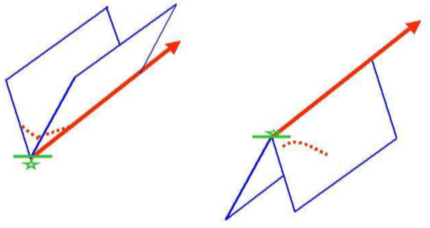Fig. 107 Deterministic Chaos. The thick line with an arrow represents the unique trajectory emerging as solution of the equations of motion from the initial condition denoted by a star. The transverse stability (left) or instability (right) means that trajectories starting from nearby initial points (dotted lines) converge to (left) or diverge from (right) the ideal trajectory. The right case is a regular motion; the left one is chaotic, with information loss.¶ {Arecchi, 2007a, Fig. 1}

Initial conditions to the left or right of the ideal one converge toward the ideal trajectory or diverge away depending on whether the transverse stability analysis yields a valley–like (left) or hill-like (right) landscape. In the second case, we loose information of the initial preparation at a rate which depends on the steepness of the down-hill. This information loss does not require interaction with a disturbing environment as in noise problems; it is just a sensitive dependence on the initial conditions nowadays called deterministic chaos. K denotes the rate of information loss, after Kolmogorov. Newton restricted his dynamics to 2-body interactions which are regular as the in the left figure. In 1890 Henry Poincaré showed that the gravitational problem with 3 or more interacting bodies displays generically the transverse instability depicted on the right.

Chaos can be controlled by additional external degrees of freedom, which change the slope of the transverse potential without perturbing the longitudinal trajectory (Fig 2).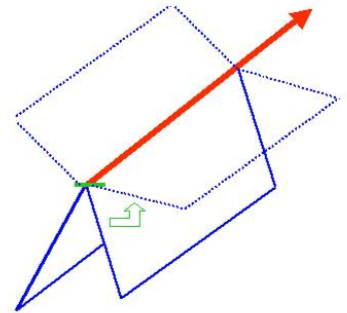Fig. 108 Adding new degrees of freedom in a suitable way, the transverse instability can be reduced (right) or wholly eliminated (left), while conserving the longitudinal trajectory The addition of extra degrees of freedom implies a change of code, thus it can be seen as a new level of description of the same physical system.¶ Control of Chaos, {Arecchi, 2007a, Fig. 2}

Changing the number of degrees of freedom amounts to changing the descriptive code. In the perceptual case, we will see in Sec. 2, that a collective neuron dynamics is in general chaotic. In presence of a specific sensory input, different top-down perturbations due to different stored memories modify the transverse stability by different amounts. There will be a competition among different interpretations, that is, different perturbations of the sensorial stimulus by past memories. The winning result is that which assures the highest stability during the perception time (usually, a perceptual window is of the order of a few hundred milliseconds). In fig.2 we depict the role of two different control perturbations. Thus, we anticipate already from Sec.2 that any coherent perception is an interpretation, that is, a change of code with respect to that imposed by the sheer sensorial stimulus.

If we do not introduce control, information on the initial setting has been lost after a time K-1 and one must re-assign the initial condition in order to predict the future: think e.g. of meteorological forecast. This may be very information consuming; that’s why a novel descriptive code, which reduces K, may be more effective than the old one.

Within the reductionistic code, problems have a monotonic C-K behavior. For K=0 we have C=0; thus it has been straightforward to design an algorithm, BACON, (Simon) which infers Kepler’s laws from the regularities of the planets’ motions.

For K → ∞ (Boltzmann gas) a dynamical description requires N → ∞ variables hence C → ∞. However, for most experimental situations a thermodynamical description is sufficient, and thermodynamics has a very low C. This means that re-coding a problem in terms of new indicators suggested by a specific experience (semiosis) replaces the old code of the microscopic description with a new one having lower complexity. This reduction is by no means a loss of resolution, since the lost bits are inaccessible and hence they could not be dubbed as hidden variables. We generalize saying that any code transformation implying a complexity reduction – as it occurs in most human endeavors, as e.g. translation of a text to another language - requires a mechanism of information loss and replacement with new information not included in the primitive code (information swapping).

On the contrary, working within a fixed code, any complexity change is due to a different organization of the system under scrutiny, as it occurs in Renormalization Group applications called Multi-Grid (Solomon). The fixed code means that the analysis can be carried by a computer; this automatic version of complexity does not match our pre-scientific expectations. We rather call it complication, leaving the concept of complexity to the different points of view under which we grasp the same system under different codes (Sec 3).

In Fig 3 we compare two different definitions of complexity-complication, namely the algorithmic one CA, already defined above and introduced by G. Chaitin in 1965 (Chaitin), and the logical depth D, introduced in 1985 by C. Bennett (Bennett) as the time necessary to execute a program starting from the shortest instruction.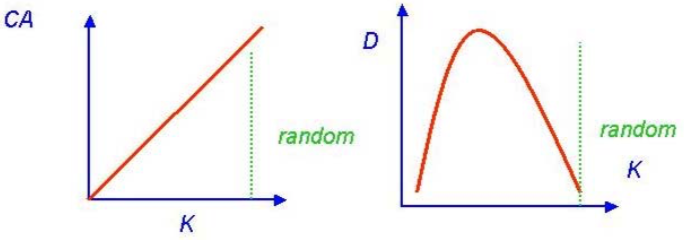Fig. 109 Left: CA=algorithmic complexity (Chaitin); it increases monotonically with Kolmogorov entropy K and is maximal for a random bit sequence. Right: D = logical depth (Bennett);it has a maximum for intermediate K and is very small for a random sequence.¶ Two definitions of complexity-complication, {Arecchi, 2007a, Fig. 2}.

For low K the two definitions are equivalent, but, while CA increases monotonically with K, D goes to zero for high K. Indeed think of a random number. CA, and K as well, will increase with the number of digits, whereas D is very short: once the number of digits has been collected in the long instruction, then the execution is just: “print it”.

As an example, let us consider the Ising model. It consists of a set of coupled spin 1⁄2 particles in a thermostat at fixed temperature. At low temperature (left), the mutual interactions prevail over the thermal disturbance and all spins will be aligned parallel in an ordered collective state with low complexity. At high temperature (right), the thermal perturbation exceeds the mutual coupling and the spins are randomly distributed, hence both CA and D will be like the right extreme of Fig.3. In the middle, at a critical temperature Tc, the two forces acting on each spin will be comparable and the whole system organizes in large clusters of parallel spins. The clusters undergo large size fluctuations; hence D reaches a maximum which scales with a power z of the system size L. In 3D, z = 0.2.

Many further measures of complexity have been introduced and a list can be found in a review volume ({Arecchi and Farini, 1996). They have a common feature, namely, since they rely on the information content of the problem under consideration, they can be considered as varieties of what we called complication. We call creativity any code change that takes place from a high C model to a lower C model. Some well-known examples are collected in the Table 1.

Table 32 Reduction of complexity by code change

Unreduced

Reduced

Electricity; magnetism; optics

Maxwell electromagnetic equations

Mendeleev table

Quantum atom (Bohr, Pauli)

Zoo of more than 100 elementary particles

SU (3)- quarks (M Gell Mann)

Scaling laws in phase transitions

Renormalization Group (K. Wilson)

## Cognition¶

{Arecchi, 2007a}

On the other hand, cognition, defined as a world view that can be formulated in a given language and shared with other agents, is considered as a higher level endeavor with respect to perception.

A cognitive agent is not susceptible of a closed physical description, since it changes its amount of information by exposure to an environment; this fact has been called bio-semiosis and it has been taken as the distinctive feature of a living system, from a single cell to humans (Sebeok). For this reason, cognitive science has been considered thus far as a territory extraneous to physics. Here we explore intersections of this area of investigation with physics, having in mind the recent progress in nonlinear dynamics and complex systems.

## Two sorts of cognition¶

{Arecchi, 2007a}

{Arecchi, 2011}

So far, we have been treating synchronization as the collective behavior of a large array of neurons, each one behaving as a chaotic dynamical system .As a fact, in a wet brain it is hard to exploit a Newtonian approach, whereby an equation establishes a functional relation F between data d and hypotheses h, that is,

$d = F(h)$

To be less model-dependent, we replace the dynamics with a statistical approach based on Bayes inference (Bayes, 1763). It consists of the following procedure.

1.  Starting from an initial situation, formulate a wide set of hypotheses h, each of which being assigned an a-priori probability P(h);

2.  Each h, inserted into a model of evolution, generates data d with the conditional probability P(d|h) that d results from h;

3. Doing a measurement, we observe a particular data d, with an associated probability P(d);

4. The combination of (iii)+(ii) selects a particular hypothesis h* that has the highest a posteriori probability among (i).

To summarize:

$P(h*) = P(h|d) = P(h) \cdot \frac{P(d|h)}{P(d)}$

Whence h* denotes the most plausible hypothesis, since it has the largest probability.

In a probability space, we can update the vantage point and repeat the procedure in a recursive way, sticking to the same algorithm corresponding to the chosen model P(d|h). It is like climbing a probability mountain, whose top represents the maximum plausibility (Arecchi 2007a,b).

The model P(d|h) is the algorithm that we provide to a computer, making it an expert system that formulates wide sets of hypotheses to be compared with the data.

We interpret an apprehension as a Bayes inference, as follows (Fig.3). Within the synchronization interval (around 1 sec) the following things occur. One has to select the most plausible hypothesis h* among a large number of h. The memory is equipped with a procedural model P(d|h) that generates a data d for each h; on the other hand, the sensorial input consists of a precise d; thus Bayes procedure selects the a posteriori h* that best fits the actual data d. In conclusion, out of a wide set of hypotheses h, the actual data d constrains the selection of the most plausible hypothesis h*, via the algorithm, or model, P(d|h). (Fig3)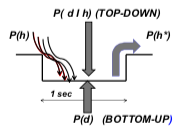Fig. 110 Apprehension as Bayes inference (h = a priori hypotheses; d = data). Selection of the a posteriori hypothesis h* starting from a manifold of h, by joint action of a sensory stimulus (bottom-up) and an interpretation model (top-down)¶ {Arecchi, 2011} Fig. 3.

Swapping model is a re-coding operation that takes place during the half-second processing that occurs between the arrival of the bottom-up stimuli and the expression of a suitable reaction in terms of motor decisions. This top-down elaboration exploits a set of models P(d|h) retrieved from the memory, selecting what the inner mechanisms (emotions, values, attention) suggest as the most appropriate. This model set is built in previous training stages in animals or inserted as instructions in robots, anyhow, the set is finite both for animals and robots.

Successive applications of the Bayes inference using the same algorithm correspond to successive apprehensions whereby we increase the plausibility of the final guess h* upon which the agent reacts (Fig.4).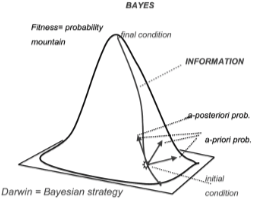Fig. 111 Successive applications of Bayes to the experiments.¶ {Arecchi, 2011} Fig. 4.

The procedure consists in climbing up the probability mountain through a steepest gradient line. Each point of the line carries information related to the local probability by Shannon formula. An investigator as Sherlock Holmes is strictly Bayesian. He has a model of the crime (algorithm) and he tests different hypotheses sets h, by comparing them at each step with collected data d and thus extracting the most plausible one h*. The process is then re-iterated in a recursive way, sticking to the same model, but with updated datasets d. Such a procedure is nowadays implemented in expert systems that assist the medical diagnosis.

However, living in a complex environment entails a change of algorithm in successive endeavors. Fig.4 can be seen as the implementation of an ecological process, whereby a cognitive agent, equipped with a world model P(d|h), interacts in a recursive way with the environment, updating its starting point. Climbing up a single slope can be automatized by a steepest gradient program. It is a non-semiotic procedure, and the corresponding algorithm can be assigned a Chaitin algorithmic complexity (Chaitin, 1987).

This does not work in a complex situation. Based on two decades of intensive debate, we can define complexity as a situation that cannot be grasped by a single model. Swapping algorithm is a non-algorithmic procedure; a complex system is one with a many-mountain probability landscape (fig.5).

Fig. 5. Semantic complexity

On the contrary, to jump to other slopes, and thus continue the Bayes strategy elsewhere, is a creativity act, implying a holistic comprehension of the surrounding world (semiosis). We call “semantically complex” the multi-mountain landscape and attribute a different meaning to each peak. It has been guessed that semiosis is the property that discriminates living beings from Turing machines (Sebeok, 1992). Here we show that a non-algorithmic procedure, that is, a jump from one Bayesian model to another is what we have called creativity. Semiosis is then equivalent to creativity, as outlined in Fig.5. The difference between a single Bayesian strategy and a creative jump is the same as the difference between normal science and paradigm shift (Kuhn, 1962)

The Gödel’s first incompleteness theorem (1931) can be considered as a creative jump in a complex landscape, as illustrated in Fig.6.

Fig. 6. From Bayes to Gödel

The theorem states that, for any consistent formal and computably enumerable theory that proves basic arithmetical truths, an arithmetical statement that is true, but not provable in the theory, can be constructed. “Provable in the theory” means “derivable from the axioms and primitive notions of the theory, using standard first-order logic”. The logical steps occurring in that statement are graphically represented in Fig.6. There is a computer equivalent of this theorem, that Turing (Turing, 1936) called the Halting problem, namely, a universal computer, for a generic input, cannot decide to stop. The jump from a model to another, guided by semiosis, is a non-algorithmic operation, peculiar of a living being in interaction with the environment. The question arises: can we foresee an evolution of the computing machines, so that they can swap algorithm by an adaptive procedure? The answer is yes within a scenario with a finite repertoire. Furthermore, the swapping is based on a variational procedure whereby the next model is just a small variation of the previous one which by itself has to be structurally stable. Such is Holland’s genetic algorithm (Holland, 1992). However the application of a selected Bayes algorithm to a complex environment can lead to instabilities, in the sense that a small variation might introduce discontinuous jumps. This requires recurring to an altogether different algorithm, that is, violating the set of rules previously stipulated. Such a non-algorithmic jump enables a creative mathematician to grasp the truth of propositions compatible with a set of axioms but not accessible through the formalism one is using; this is the 1931 Gödel theorem (fig.6). We do not see how a machine can violate the plan upon which it has been designed, going well beyond the variational changes allowed by a genetic algorithm strategy.

[MORE ON JUDGMENT]